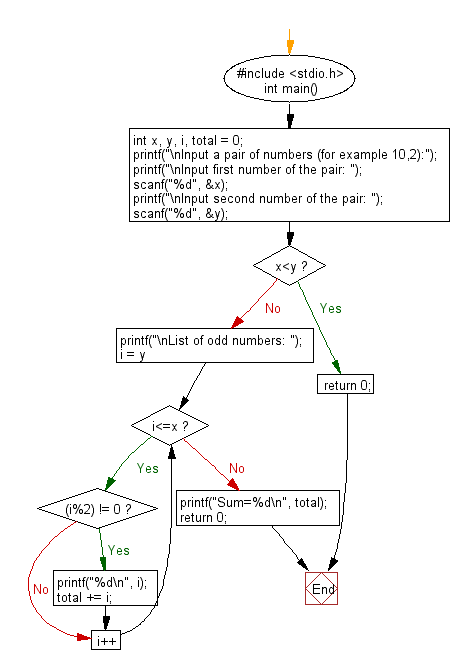﻿ C : Sum consecutive odd numbers from a pair of integers

# C Exercises: Compute the sum of consecutive odd numbers from a given pair of integers

## C Basic Declarations and Expressions: Exercise-34 with Solution

Write a C program to compute the sum of consecutive odd numbers from a given pair of integers.

Sample Solution:

C Code:

``````#include <stdio.h>
int main() {
int x, y, i, total = 0; // Declare variables for user input and calculations
printf("\nInput a pair of numbers (for example 10,2):");
printf("\nInput first number of the pair: ");
scanf("%d", &x); // Read the first number
printf("\nInput second number of the pair: ");
scanf("%d", &y); // Read the second number

if (x < y) {
return 0; // If x is less than y, exit the program
}

printf("\nList of odd numbers: ");
for (i = y; i <= x; i++) {
if ((i % 2) != 0) {
printf("%d\n", i); // Print odd numbers within the range
total += i; // Add odd numbers to the total
}
}

printf("Sum=%d\n", total); // Print the total sum

return 0;
}
``````

Sample Output:

```Input a pair of numbers (for example 10,2):
Input first number of the pair: 10

Input second number of the pair: 2

List of odd numbers: 3
5
7
9
Sum=24
```

Flowchart:C programming Code Editor:

What is the difficulty level of this exercise?

Test your Programming skills with w3resource's quiz.

﻿